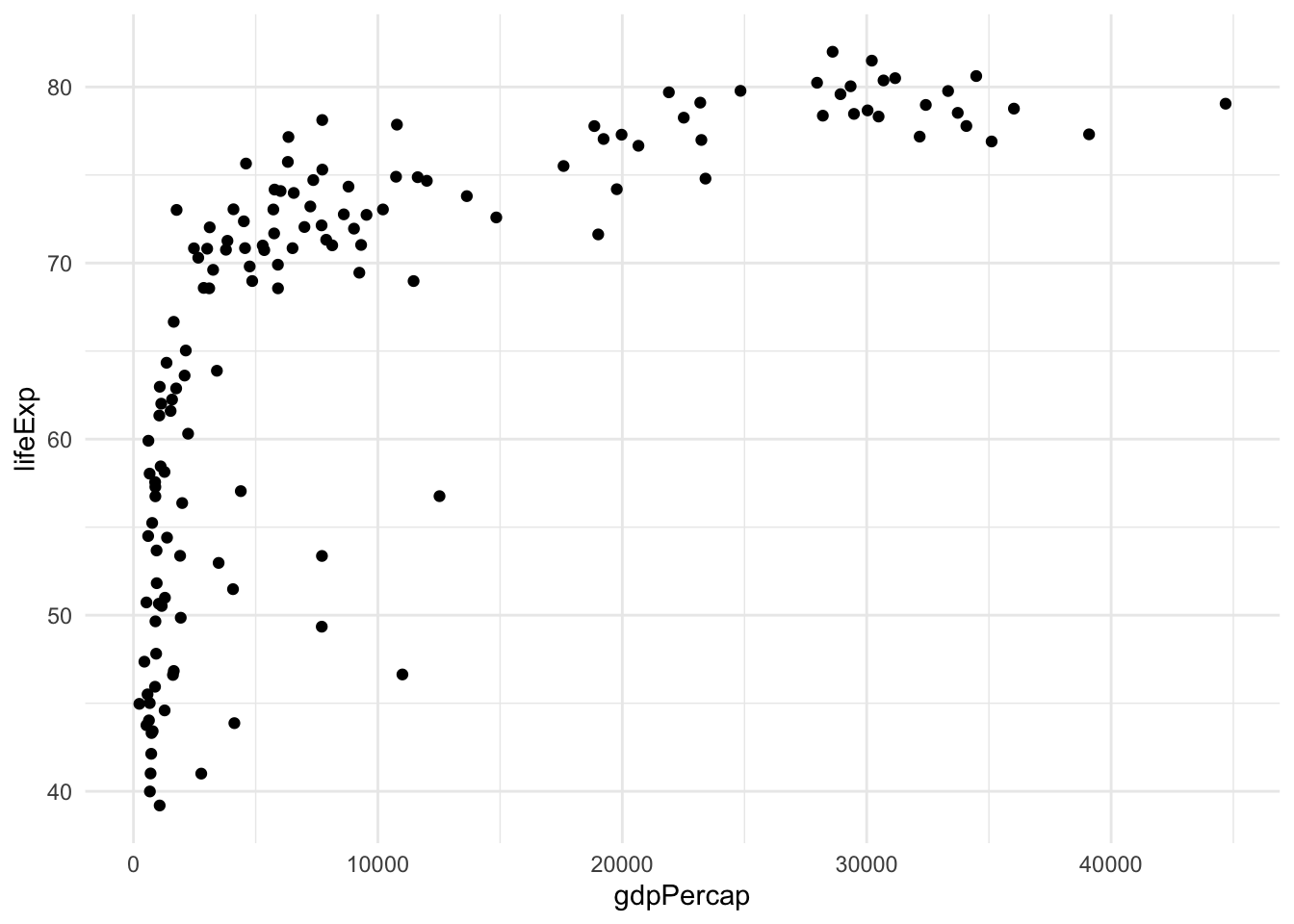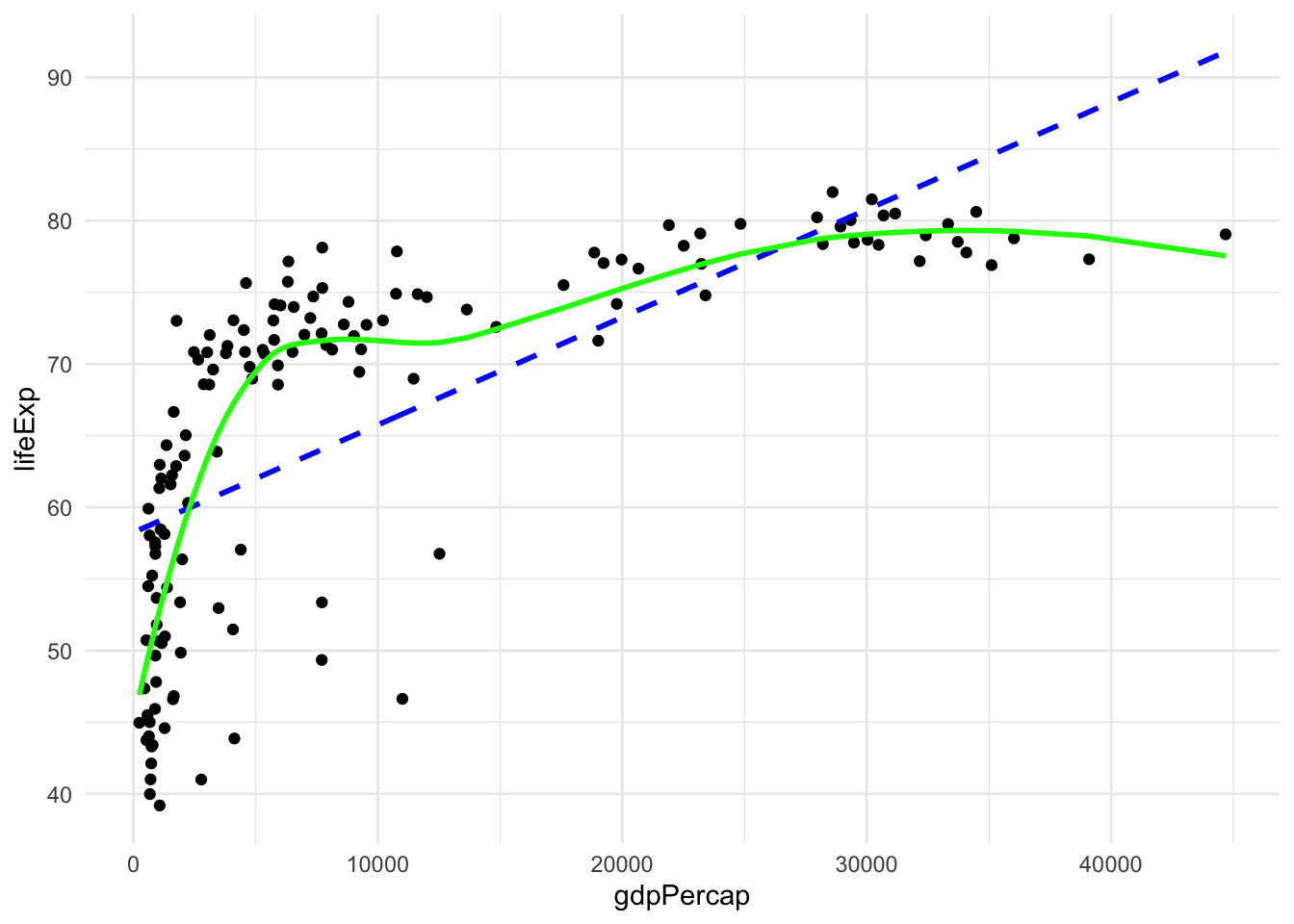## Repeating the gapminder analysis

Let’s start easy by just repeating some steps but with data from a different year.

### Create the gapminder scatterplot using data from the year 2002

gm2002 <- gapminder %>%
filter(year == 2002)
gm_scatterplot <-
ggplot(gm2002,
aes(x = gdpPercap, y = lifeExp)) +
geom_point()
gm_scatterplot#### Create an lm model to predict lifeExp

model_lm <- lm(lifeExp ~ gdpPercap, data = gm2002)
predictions_lm <- augment(model_lm)

#### Create a loess model to predict lifeExp

model_loess <- loess(lifeExp ~ gdpPercap, span = .75, data = gm2002)
predictions_loess <- augment(model_loess)

#### Plot showing the two models

gm_scatterplot +
geom_line(data = predictions_lm, size = 1,
color = "blue",
linetype = "dashed",
aes(y = .fitted)) +
geom_line(data = predictions_loess, size = 1,
color = "green",
aes(y = .fitted))mean(residuals(model_lm)^2)
##  80.11716
mean(residuals(model_loess)^2)
##  45.21583

## Predicting on new data

Models are supposed to capture/use structure in the data that corresponds to structure in the real world. And if the real world isn’t misbehaving, that structure should be somewhat stable.

For example, suppose the relationship changed dramatically from one time period to another time period. Then it would be less useful/interesting to have a model fit on data at one time period, because the same model might have a poor fit on data from a different time period.

Let’s explore this with our gapminder models

#### Predictions on different years

Create datasets for the desired years

gm2007 <- gapminder %>% filter(year == 2007)
gm1997 <- gapminder %>% filter(year == 1997) 

Predict using newdata argument, then pull the residuals from the resulting data.frame

lm_resid2007 <- augment(model_lm, newdata = gm2007) %>%
pull(.resid)
lm_resid1997 <- augment(model_lm, newdata = gm1997) %>%
pull(.resid)
loess_resid2007 <- augment(model_loess, newdata = gm2007) %>%
pull(.resid)
loess_resid1997 <- augment(model_loess, newdata = gm1997) %>%
pull(.resid)

#### Check 1997

mean(lm_resid1997^2)
##  67.23804
mean(loess_resid1997^2)
##  31.56738

#### Check 2007

mean(lm_resid2007^2)
##  80.1876
mean(loess_resid2007^2, na.rm = TRUE)
##  49.54716

One trade-off we see here: the loess function does not have any default way of extrapolating to observations outside the range of the original data (values of gdpPercap in 2007 that are larger than the maximum in 2002).

## Conclusion/notes

The more complex, loess model performs better than the linear model even when tested on data from 5 years earlier or later.

Sometimes a more complex model really is better!

Question: Can we break it? Let’s change the span parameter in the loess function to make it even more complex and see if we keep reaching the same conclusion.

Answer: Even after decreasing to span = 0.1 the more complex model was still better!

Question: How can we change this setup so that the linear model isn’t always worse?

Answer: Try a logarithmic transformation on the gdpPercap variable to improve the fit of the linear model first, then maybe the linear model will do better on data from a different year than loess with a low span value.

gm2002 <- gapminder %>%
filter(year == 2002) %>%
mutate(log_gdpPercap = log10(gdpPercap))
gm2007 <- gapminder %>% filter(year == 2007) %>%
mutate(log_gdpPercap = log10(gdpPercap))
gm1997 <- gapminder %>% filter(year == 1997) %>%
mutate(log_gdpPercap = log10(gdpPercap))

Now repeat other code changing gdpPercap to log_gdpPercap.

It seems that:

• More complex models (almost) always have lower MSE when the errors are computed on the same data as the model fitting function
• More complex models can also have lower MSE when the errors are computed on new data that the model fitting function did not access
• But, sometimes simpler models have lower MSE on new data